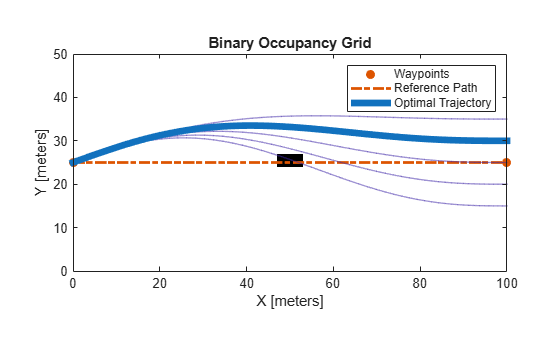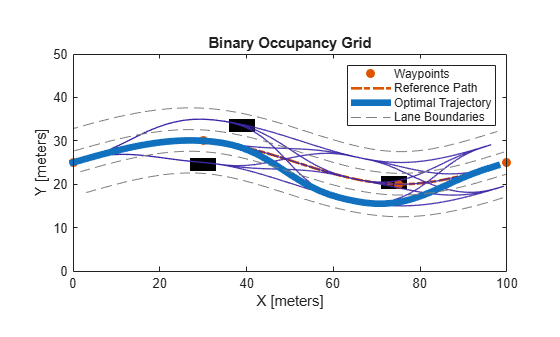# trajectoryOptimalFrenet

Find optimal trajectory along reference path

## Description

The `trajectoryOptimalFrenet` object is a path planner which samples and evaluates local trajectories based on a reference path. The planner generates a set of terminal states based on the reference path and other parameters in the object. The planner then connects the state to each terminal state using 4th or 5th order polynomials. To choose an optimal path, sampled trajectories are evaluated for kinematic feasibility, collision, and cost.

## Creation

### Syntax

``trajectoryOptimalFrenet(refPath,validator)``
``planner = trajectoryOptimalFrenet(___,Name,Value)``

### Description

````trajectoryOptimalFrenet(refPath,validator)` creates a `trajectoryOptimalFrenet` object with reference path, `refPath`, in the form of an n-by-2 array of ```[x y]``` waypoints and a state validator, `validator`, specified as a `validatorOccupancyMap` object.```

example

````planner = trajectoryOptimalFrenet(___,Name,Value)` sets additional properties using one or more name-value pairs in any order.```

### Input Arguments

expand all

Reference path, specified as an n-by-2 matrix of ```[x y]``` pairs, where n is the number of waypoints.

Example: [100,100;400,400]

Data Types: `double`

State validator object, specified as a `validatorOccupancyMap` object.

## Properties

expand all

Note

For the `'Weights'` and `'FeasibilityParameters'` properties, you cannot specify the entire structures at once. Instead, set their fields individually as name-value pairs. For example, `trajectoryOptimalFrenet(refPath,validator,'Deviation',0)` sets the `'Deviation'` field of the structure `'Weights'`.

The weights for all trajectory costs, specified as a structure containing scalars for the cost multipliers of the corresponding trajectory attributes. The total trajectory cost is a sum of all attributes multiplied by their weights. The structure has the these fields.

The cost function multiplies the weight by the total time taken to reach the terminal state. Specify this value as the comma-separated pair of `'Time'` and a positive scalar in seconds.

Data Types: `double`

The cost function multiplies the weight by the total length of the generated trajectories. Specify this value as the comma-separated pair of `'ArcLength'` and a positive scalar in meters.

Data Types: `double`

The cost function multiplies the weight by the integral of lateral jerk squared. This value determines the aggressiveness of the trajectory in the lateral direction (perpendicular to the reference path). Specify this value as the comma-separated pair of `'LateralSmoothness'` and a positive scalar. To penalize lateral jerk in the planned trajectory increase this cost value.

Data Types: `double`

The cost function multiplies the weight by the integral of longitudinal jerk squared. This value determines the aggressiveness of the trajectories in the longitudinal direction (direction of the reference path). Specify this value as the comma-separated pair of `'LongitudinalSmoothness'` and a positive scalar. To penalize large change in forward and backward acceleration increase this cost value.

Data Types: `double`

The cost function multiplies the weight by the perpendicular distance from the reference path at the end of the trajectory in meters. Specify this value as the comma-separated pair of `'Deviation'` and a positive scalar in meters.

Data Types: `double`

Data Types: `struct`

Feasibility parameters, specified as a structure containing scalar values to check the validity of a trajectory. The structure has the these fields.

Maximum curvature that the vehicle can execute. Specify this value as the comma-separated pair of `'MaxCurvature'` and a positive real scalar in m-1. This value determines the kinematic feasibility of the trajectory.

Data Types: `double`

Maximum acceleration in the direction of motion of the vehicle. Specify this value as the comma-separated pair of `'MaxAcceleration'` and a positive real scalar in m/s2. To lower the limit on the acceleration of the vehicle in the forward or reverse direction decrease this value.

Data Types: `double`

Data Types: `struct`

Time interval between discretized states of the trajectory. Specify this value as the comma-separated pair of `'TimeResolution'` and a positive real scalar in seconds. These discretized states determine state validity and cost function.

Data Types: `double`

The user-defined cost function, specified as a function handle. The function must accept a matrix of n-by-7 states, `TRAJSTATES`, for each trajectory and return a cost value as a scalar. The `plan` function returns the path with the lowest cost.

For example, ```leftLaneChangeCost = @(states)((states(end,2) < refPath(end,2))*10)``` creates a cost function handle to prioritize left lane changes.

Data Types: `function handle`

The `'TrajectoryList'` property, returned as a structure array of all the candidate trajectories and their corresponding parameters. Each structure has the these fields:

• `Trajectory` — An n-by-7 matrix of ```[x, y, theta, kappa, speed, acceleration, time]```, where n is the number of trajectory waypoints.

• `Cost` — Cost of the trajectory.

• `MaxAcceleration` — Maximum acceleration of the trajectory.

• `MaxCurvature` — Maximum curvature of the trajectory.

• `Feasible` — A four-element vector ```[velocity, acceleration, curvature, collision]``` indicating the validity of the trajectory.

The value of the elements can be either,

• `1` — The trajectory is valid.

• `0` — The trajectory is invalid.

• `-1` — The trajectory is not checked.

Data Types: `struct`

A structure that contains a list of goal states relative to the reference path. These parameters define the sampling behavior for generating alternative trajectory segments between start and each goal state. The structure has the these fields.

Lengths of the trajectory segment, specified as a vector in meters.

Data Types: `double`

Array of deviations from reference path in perpendicular direction at goal state, specified as a vector in meters.

Data Types: `double`

Velocity at the goal state in the direction of motion, specified as a positive scalar in m/s.

Data Types: `double`

Acceleration at the goal state in the direction of motion, specified as a positive scalar in m/s2.

Data Types: `double`

Array of end-times for executing the trajectory segment, specified as a positive vector in seconds.

Data Types: `double`

Data Types: `struct`

Waypoints of reference path, specified as an n-by-2 matrix of ```[x y]``` pairs, where n is the number of waypoints. Waypoints act as a reference for planning alternative trajectories optimized by this planner.

Data Types: `double`

Number of longitudinal segments for each trajectory. Specify this value as the comma-separated pair of `'NumSegments'` and a positive scalar. This property generates intermediate longitudinal terminal states to which all lateral terminal states are combined with for generating more motion primitives to each terminal state.

For example, `'NumSegments',2` creates two partitions between each longitudinal terminal state. Trajectories are generated to reach the intermediate longitudinal states with all the available lateral terminal states.

Data Types: `double`

Deviation offset from the reference path in the lateral direction. Specify this value as the comma-separated pair of `'DeviationOffset'` and a scalar. A negative value offset the deviation to the right, and a positive value offset the deviation to the left of the reference path in the lateral direction. Set this property to bias your solution to a certain turn direction when avoiding obstacles in the reference path.

Data Types: `double`

## Object Functions

 `cart2frenet` Convert Cartesian states to Frenet states `copy` Create deep copy of object `frenet2cart` Convert Frenet states to Cartesian states `plan` Plan optimal trajectory `show` Visualize trajectories

## Examples

collapse all

This example shows how to plan an optimal trajectory using a `trajectoryOptimalFrenet` object.

Create and Assign Map to State Validator

Create a state validator object for collision checking.

`stateValidator = validatorOccupancyMap;`

Create an obstacle grid map.

```grid = zeros(50,100); grid(24:26,48:53) = 1;```

Create a `binaryOccupancyMap` with the grid map.

`map = binaryOccupancyMap(grid);`

Assign the map and the state bounds to the state validator.

```stateValidator.Map = map; stateValidator.StateSpace.StateBounds(1:2,:) = [map.XWorldLimits; map.YWorldLimits];```

Plan and Visualize Trajectory

Create a reference path for the planner to follow.

`refPath = [0,25;100,25];`

Initialize the planner object with the reference path, and the state validator.

`planner = trajectoryOptimalFrenet(refPath,stateValidator);`

Assign longitudinal terminal state, lateral deviation, and maximum acceleration values.

```planner.TerminalStates.Longitudinal = 100; planner.TerminalStates.Lateral = -10:5:10; planner.FeasibilityParameters.MaxAcceleration = 10;```

Specify the deviation offset value close to the left lateral terminal state to prioritize left lane changes.

`planner.DeviationOffset = 5;`

Trajectory Planning

Initial cartesian state of vehicle.

`initCartState = [0 25 pi/9 0 0 0];`

Convert cartesian state of vehicle to Frenet state.

`initFrenetState = cart2frenet(planner,initCartState);`

Plan a trajectory from initial Frenet state.

`plan(planner,initFrenetState);`

Trajectory Visualization

Visualize the map and the trajectories.

```show(map) hold on show(planner,'Trajectory','all')```This example shows how to partition the longitudinal terminal states in optimal trajectory planning using a `trajectoryOptimalFrenet` object.

Create and Assign Map to State Validator

Create a state validator object for collision checking.

`stateValidator = validatorOccupancyMap; `

Create an obstacle grid map.

```grid = zeros(50,100); grid(25:27,28:33) = 1; grid(16:18,37:42) = 1; grid(29:31,72:77) = 1;```

Create a `binaryOccupancyMap` with the grid map.

`map = binaryOccupancyMap(grid);`

Assign the map and the state bounds to the state validator.

```stateValidator.Map = map; stateValidator.StateSpace.StateBounds(1:2,:) = [map.XWorldLimits; map.YWorldLimits];```

Plan and Visualize Trajectory

Create a reference path for the planner to follow.

`refPath = [0,25;30,30;75,20;100,25];`

Initialize the planner object with the reference path, and the state validator.

`planner = trajectoryOptimalFrenet(refPath,stateValidator);`

Assign longitudinal terminal state, lateral deviation, and maximum acceleration values.

```planner.TerminalStates.Longitudinal = 100; planner.TerminalStates.Lateral = -5:5:5; planner.FeasibilityParameters.MaxAcceleration = 10;```

Assign the number of partitions for the longitudinal terminal state.

`planner.NumSegments = 3;`

Trajectory Planning

Initial Frenet state of vehicle.

`initFrenetState = zeros(1,6);`

Plan a trajectory from initial Frenet state.

`plan(planner,initFrenetState);`

Trajectory Visualization

Visualize the map and the trajectories.

```show(map) hold on show(planner,'Trajectory','all') hold on```

Generate Lane Boundaries

Calculate end of reference path as Frenet state.

`refPathEnd = cart2frenet(planner,[planner.Waypoints(end,:) 0 0 0 0]);`

Calculate lane offsets on both sides of the lateral terminal states with half lane width value.

`laneOffsets = unique([planner.TerminalStates.Lateral+2.5 planner.TerminalStates.Lateral-2.5]);`

Calculate positions of lanes in Cartesian state.

```numLaneOffsets = numel(laneOffsets); xRefPathEnd = ceil(refPathEnd(1)); laneXY = zeros((numLaneOffsets*xRefPathEnd)+numLaneOffsets,2); xIndex = 0; for laneID = 1:numLaneOffsets for x = 1:xRefPathEnd laneCart = frenet2cart(planner,[x 0 0 laneOffsets(laneID) 0 0]); xIndex = xIndex + 1; laneXY(xIndex,:) = laneCart(1:2); end xIndex = xIndex + 1; laneXY(xIndex,:) = NaN(1,2); end```

Plot lane boundaries.

`plot(laneXY(:,1),laneXY(:,2),'LineWidth',0.5,'Color',[0.5 0.5 0.5],'DisplayName','Lane Boundaries','LineStyle','--')`## Limitations

• Self-intersections in the reference path can lead to unexpected behavior.

• The planner does not support reverse driving.

• Initial orientation for planning should be within `-pi/2` and `pi/2` to the reference path.

• Limit the number of TerminalStates for real-time applications since computational complexity grows with it.

 Werling, Moritz, Julius Ziegler, Sören Kammel, and Sebastian Thrun. "Optimal Trajectory Generation for Dynamic Street Scenarios in a Frenet Frame." 2010 IEEE International Conference on Robotics and Automation. 2010, pp. 987–993.